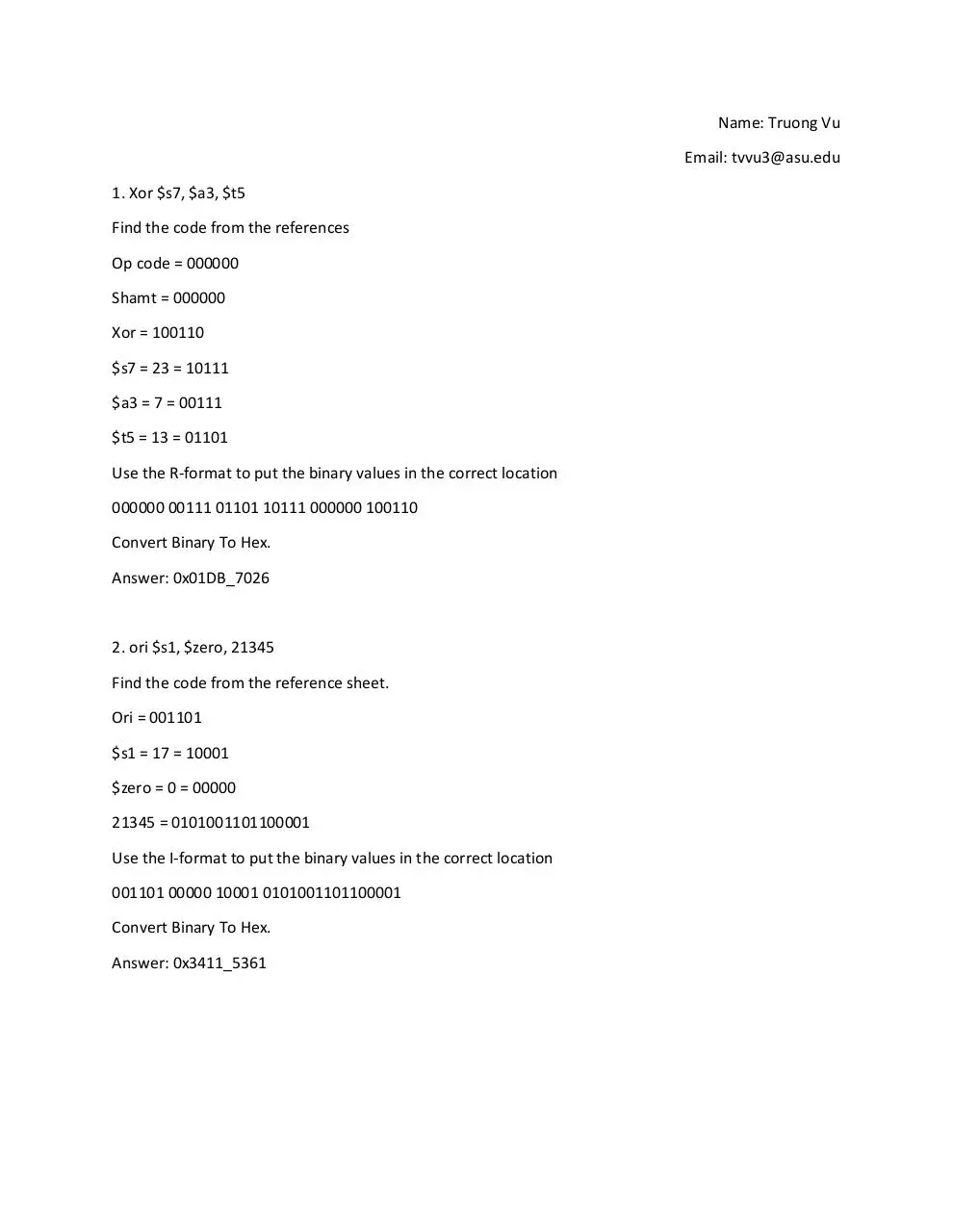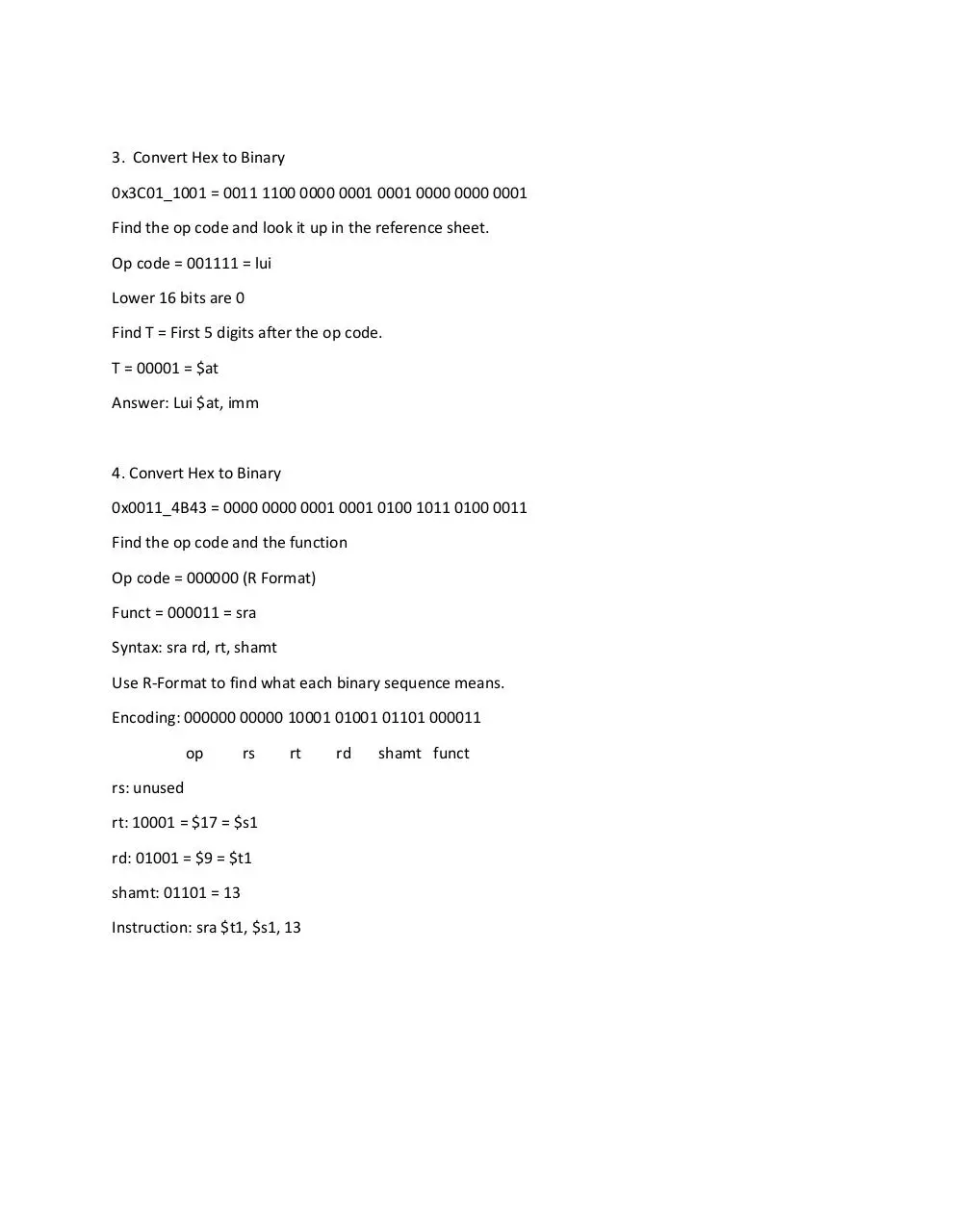# 230 s15 h03 tvvu3 .pdf

### File information

Author: Truong

This PDF 1.5 document has been generated by MicrosoftÂ® Word 2013, and has been sent on pdf-archive.com on 17/04/2015 at 20:55, from IP address 68.104.x.x. The current document download page has been viewed 808 times.
File size: 100.95 KB (2 pages).
Privacy: public file

### Document preview

Name: Truong Vu
Email: tvvu3@asu.edu
1. Xor \$s7, \$a3, \$t5
Find the code from the references
Op code = 000000
Shamt = 000000
Xor = 100110
\$s7 = 23 = 10111
\$a3 = 7 = 00111
\$t5 = 13 = 01101
Use the R-format to put the binary values in the correct location
000000 00111 01101 10111 000000 100110
Convert Binary To Hex.

2. ori \$s1, \$zero, 21345
Find the code from the reference sheet.
Ori = 001101
\$s1 = 17 = 10001
\$zero = 0 = 00000
21345 = 0101001101100001
Use the I-format to put the binary values in the correct location
001101 00000 10001 0101001101100001
Convert Binary To Hex.

3. Convert Hex to Binary
0x3C01_1001 = 0011 1100 0000 0001 0001 0000 0000 0001
Find the op code and look it up in the reference sheet.
Op code = 001111 = lui
Lower 16 bits are 0
Find T = First 5 digits after the op code.
T = 00001 = \$at

4. Convert Hex to Binary
0x0011_4B43 = 0000 0000 0001 0001 0100 1011 0100 0011
Find the op code and the function
Op code = 000000 (R Format)
Funct = 000011 = sra
Syntax: sra rd, rt, shamt
Use R-Format to find what each binary sequence means.
Encoding: 000000 00000 10001 01001 01101 000011
op

rs

rt

rs: unused
rt: 10001 = \$17 = \$s1
rd: 01001 = \$9 = \$t1
shamt: 01101 = 13
Instruction: sra \$t1, \$s1, 13

rd

shamt funct230-s15-h03-tvvu3.pdf (PDF, 100.95 KB)

### Share on social networks

#### HTML Code

Copy the following HTML code to share your document on a Website or Blog